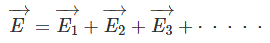Categories

## Electric field intensity lines of equipotential

Another important element of the electric field is the what is often called the electric field intensity. It is possible to diagrammatically represent the field intensity as equipotential lines on a diagram. These lines can be likened to the contour lines on a map. The equipotential lines are always perpendicular to the electric field.

It is also possible to represent these lines in three dimensions as elliptical spheroids of equal potential.

For a single point charge, the electric field intensity or potential gradient will be highest close to the charge, decaying as it moves further away.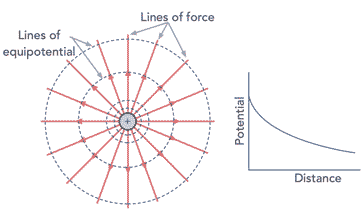Electric field contours of equipotential around a point charge

In terms of moving along these lines or elliptical spheroids of equal potential, any movement requires no work as a result of movement against the electric field because this movement is always perpendicular to the electric field.

The electric field intensity can be gauged from the closeness of the lines in a similar way to the fact that close contour lines on a map indicate a very steep incline.Lightning strike represents a large discharge between positive and negative areas of chargePhoto taken from top of Petronas Towers in Kuala Lumpur Malaysia

When the electric field intensity builds up to a sufficient degree, the dielectric between the negative and positive areas of charge can even lead to a breakdown and current will flow between them giving sparks. Small sparks are relatively commonly seen around the home, but much larger ones occur in the form of lightning.

Electric fields and Coulomb’s law are important in many aspects of electrical and electronics technology. Although the calculations may not be performed that often, a basic knowledge and understanding of the technology is very important. It enables the underlying reasons for some phenomena and elements of items like electronic components to be understood more fully.

Categories

## Electric field lines of force

An electric field is a vector quantity and can be visualised as arrows going toward or away from charges. The lines are defined as pointing radially outward, away from a positive charge, or radially inward, toward a negative charge.

The lines represent the lines of force and are often called the electric field lines. They are typically drawn as a pattern of several lines extending from the charge.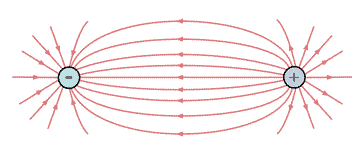Electric field lines of force between a negative and positive charge

The electric field lines point in the direction that a positive charge would accelerate if it were placed upon the line. This means that the lines are directed away from positively charged source charges and toward negatively charged source charges. To indicate these directions the electric field lines include an arrowhead that points in the appropriate direction.

In theory the pattern for an electric field would consist of an infinite number of lines. In practice, this would make any diagram unreadable, so in the interest of clarity the number of lines is usually limited. Normally just a few lines around a charge to indicate the electric field is sufficient to show the nature of the electric field in the space around the charge.

Categories

## Coulomb’s Law:

Coulomb’s Law states that like charges repel and opposite charges attract with a force proportional to the product of the charges and inversely proportional to the square of the distance between them.

Despite the simplicity of the statement of Coulomb’s Law, it is far reaching in its application, and it describes many aspects of electric fields: what they are; how they react and the like.

To understand Coulomb’s Law and the interaction of electric charges and the resulting electric field a little more, it is possible to look at the basic concepts first and then building up from this.

It is found that an electric charge has an associated electric field and this interacts with other changes with their electric fields.

In terms fo the forces it is found that the electric force is greater if the strength of the charges is greater, i.e. charges are greater. It is also greater if the charges are closer together, and decrease if they are further away from each other. Specifically, the electric force is inversely proportional to the square of the distance between the two charges, i.e. it is an “inverse square law.”

Often the electric field lines are shown in diagrams and these indicate the electric field present at any given location.

## Coulomb’s Law formula

It is often necessary to be able to express laws such as Coulomb’s Law in the form of a formula or equation.

This enables a greater understanding of the law and it also enables various calculations to be made.

F = k  Q1 Q2 / d2

Where:
F = force between the two charged objects in Newtons
Q1 = charge on object 1 in coulombs
Q2 = charge on object 2 in coulombs
k = Coulomb’s law constant. The value of this constant is depends on the medium in which the charged objects are immersed. For air the value is approximately 9.0 x 109 N • m2 / C2.
d = distance between two objects in metres.

It is worth noting that Coulomb’s law provides an accurate description of the force between two objects that can be considered as point charges. Fortunately a charged conducting sphere acts with other charged objects as though all of its charge were located at its centre, and hence it can be considered as a point charge.

As Coulomb’s law applies to point charges, the distance d in the equation above should be measured between the centres of the spheres for both objects. It is not the distance between the surfaces of the spheres. In some cases there may not bae a large difference between the two distances, but in others there will be. It is also important because the distance value is squared in the equation, and hence any discrepancies will be magnified in terms of the effect on the overall value of force.

Categories

## What is an electric field

It is probably worth starting to look at what an electric field is by defining what it is and then explaining how it all occurs and the effects of it.

### Electric field definition:

An electric field results from each point in space where charge is present in any form. The magnitude and direction of the electric field are expressed by the value of E, called electric field strength which is often referred to as the electric field, or sometimes the electric field intensity.

The electric fields, charges and forces are explained by Coulomb’s Law. As the name indicated, Coulomb’s Law was developed by Charles-Augustin de Coulomb, a French Physicist. In 1785 he published three papers about electricity and magnetism and thin them he stated his law.

The development of Coulomb’s Law was a major milestone in the development of the theory of electromagnetism, and one that was sued as the foundations for many more discoveries in later years.

Categories

## What is an Electric Field & Coulomb’s Law

Electric fields are everywhere and they can play a significant part in everyday life and science.

One of the most common examples seen of the existence of electric fields and their effects can be seen when a balloon is rubbed against a sweater and the balloon becomes charged and electric field is generated.

As a result of charge and the fact that like charges repel and unlike ones attract, the balloon can stick to walls. It is the electric field associated with the charge that enables the charges to repel and attract each other.

The basics of electric fields and their impact on items like electronic components and various electrical items can be quite important, so an understanding can not only be useful from having a wider view of electrical phenomena, but also from a practical viewpoints.

Categories

## Electric field

Coulomb’s Law describes forces acting at a distance between two charges. We can reformulate the problem by breaking it into two distinct steps, using the concept of an electric field.

• Think of one charge as producing an electric field everywhere in space.
• The force on another charge introduced into the electric field of the first, is caused by the electric field at the location of the introduced charge.

If all charges are static, you get exactly the same answers with electric field as you do using Coulomb’s Law. So, is this going to be just an exercise in clever notation? No. The electric field concept comes into its own when charges are allowed to move relative to each other. Experiments show that only by considering the electric field as a property of space that propagates at a finite speed (the speed of light), can we account for the observed forces on charges in relative motion. The electric field concept is also essential for understanding a self-propagating electromagnetic wave such as light. The electric field concept gives us a way to describe how starlight travels through vast distances of empty space to reach our eyes.If the idea of force “acting at a distance” in Coulomb’s Law seems troublesome, perhaps the idea of force caused by an electric field eases your discomfort somewhat. On the other hand, you might also question if an electric field is any more “real”. The reality of an electric field is a topic for philosophers. In any case, real or not, the notion of an electric field turns out to be useful for predicting what happens to charge.We introduce electric field initially with static charges to ease into the concept and get practice with the method of analysis.

Categories

## Electric Field Strength

Electric field strength or electric field intensity is the synonym of electric field. Electric field strength can be determined by Coulomb’s law. According to this law, the force ‘F’ between two point charges having charge Q1 and Q2 Coulombs and placed at a distance d meter from each other is given by,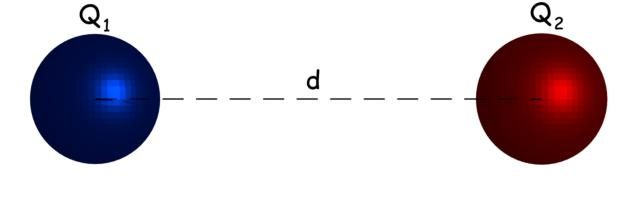Here, εo is the permittivity of vacuum = 8.854 × 10 – 12 F/m and εr is the relative permittivity of the surrounding medium.

Now, let us put Q2 = + 1 Coulomb and let us denote force F by E in the equation (1), and by doing these we get,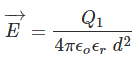This equation shows the force acting the a unit positive charge placed at a distance d from charge Q1.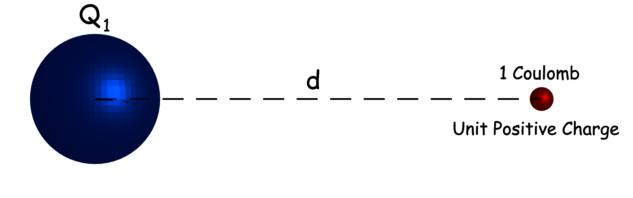As per definition, this is nothing but of electric field strength of charge Q1 at a distance d from that charge. Now, we got the expression of electric field strength or intensity. Now, by combining this expression with equation (1), we get,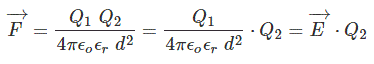The above expression shows that, if we place a charge at any point in an electric field, the product of the electric field strength at that point and the charge of the body gives the force acting on the body at that point in the field. The above expression can also be rewritten as,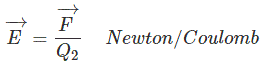Depending on this expression, the electric field strength can be expressed in Newton / Coulomb. That is unit of electric field strength is Newton / Coulomb. The electric field strength has direction and hence it is a vector quantity. Intensity means the magnitude or amount. Now field intensity similarly means the magnitude of the strength of the field. Finally electric field intensity or strength can be written as,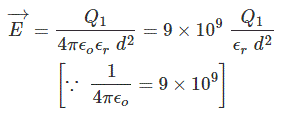So far we have discussed the electric field intensity at a point due to the influence of a single charge, but there may be a case where the point is under the filed of many charged bodies. In that case, we first have to calculate, the electric field strength at that point for individual charges and then we have to vectorially add up all the field strengths to get resultant field strength at that point.